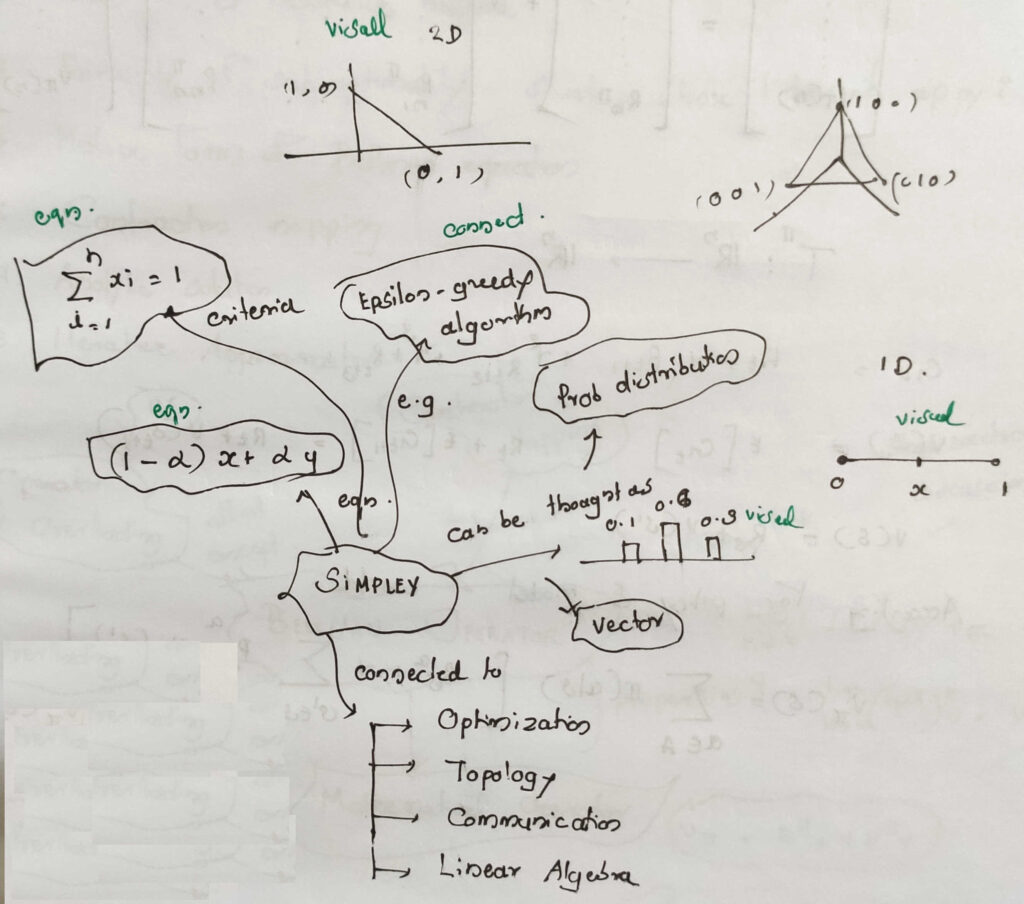# Simplex explained in one minute

The concept of simplex appears in science and engineering over and over again.

For example it appears in optimization, information theory, communication systems, linear algebra, etc. Let’s have a quick look at it from different contexts.

In general, simplex can be thought of as a mathematical expression of $\alpha x + (1-\alpha)y$. It can be a list of numbers that are between 0 and 1, all of which sum to 1.

The example shown is a specific one with only two components, but it can be generalized to having k components, called a $k$-simplex.

You might be already familiar with one form of it. The discrete probability distributions are simplex.

Depending on the levels of values its $x$-axis takes, it will have that many components all adding to 1.

The one thing to note here is that in simplex as the value of one item goes high, the magnitude of other components goes down as the sum of all components has to be unit value.

The epsilon-greedy algorithm in reinforcement learning uses a 2-simplex. The one component of it represents the percentage/fraction of time it will explore new options and the other component representing the fraction of time it will be leveraging the best option known to the algorithm so far. Just like you will occasionally try food from new restaurants while most of the time going to your favorite one.

The major domains where the concept of simplex appears include but not limited to optimization, probability theory, reinforcement learning, linear algebra, topology, information theory, etc,.

We will look at the concept from information theory perspective in another post.

Having a general perception of a concept is helpful so that you can connect these and might be even possible to use the properties of it in different situations. We will see the deeper use of simplex’s in another post.